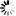# Publications in area "Mathematical Modeling & Simulation"

All || By Area || By Year

Title Published SHARCNET Authors Area
21. 2007 A.T. Lawniczak, P. Lio, S. Xie and J. Xu Mathematical Modeling & Simulation
22. 2007 A.T. Lawniczak, P. Lio, S. Xie and J. Xu Mathematical Modeling & Simulation
23. 2007 C.T. Bauch, A.M. Anonychuk, B.Z. Pham, V. Gilca, B. Duval, M.D. Krahn Mathematical Modeling & Simulation
24. 2007 A.T. Lawniczak and X. Tang Mathematical Modeling & Simulation
25. 2007 H. FukÅ Mathematical Modeling & Simulation
26. 2007 N. Harvey, A. Reeves, M.A. Schoenbaum, F.J. Zagmutt-Vergara, C. DubÃ©, A.E. Hill, B.A. Corso, B. McNab, C.I. Cartwright, M.D. Salman Mathematical Modeling & Simulation
27. 2006 A.S.R.S. Rao, B. Pham, M. Chen, A. Tricco, V. Gilca, B. Duval, M. Krahn, C.T. Bauch Mathematical Modeling & Simulation
28. 2006 A.T. Lawniczak and X. Tang Mathematical Modeling & Simulation
29. 2006 A.T. Lawniczak and X. Tang Mathematical Modeling & Simulation
30. 2006 A.T. Lawniczak and X. Tang Mathematical Modeling & Simulation
31. 2005 A.T. Lawniczak, A. Gerisch and B. Di Stefano Mathematical Modeling & Simulation
32. 2005 A.T. Lawniczak, A. Gerisch, K.P. Maxie and B. Di Stefano Mathematical Modeling & Simulation
33. 2004 H. FukÅ Mathematical Modeling & Simulation
34. 2004 A.T. Lawniczak, K.P. Maxie and A. Gerisch Mathematical Modeling & Simulation
35. 2004 A.T. Lawniczak, K.P. Maxie and A. Gerisch Mathematical Modeling & Simulation
36. 2004 K.P. Maxie, A.T. Lawniczak and A. Gerisch Mathematical Modeling & Simulation
37. 2004 K.P. Maxie, A.T. Lawniczak and A. Gerisch Mathematical Modeling & Simulation
38. 2004 A.T. Lawniczak, K. P. Maxie and A. Gerisch Mathematical Modeling & Simulation
39. 2003 C.T. Bauch and D.J.D. Earn Mathematical Modeling & Simulation
40. 2003 C.T. Bauch and D.J.D. Earn Mathematical Modeling & Simulation
Back to all areas